# Wind Speed Measurements

Average daily wind speed at 12 meteorological stations in the Republic of Ireland 1961-1978

Predict average daily wind speed at Malin Head Weather Station from 11 other meteorological stations in the Republic of Ireland. These data were originally collected in order to assess whether wind energy was a viable option in the Republic of Ireland.

## Examples

### Basic Examples

Retrieve the resource:

 In:=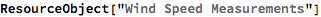Out=Retrieve the default content:

 In:=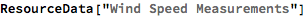Out=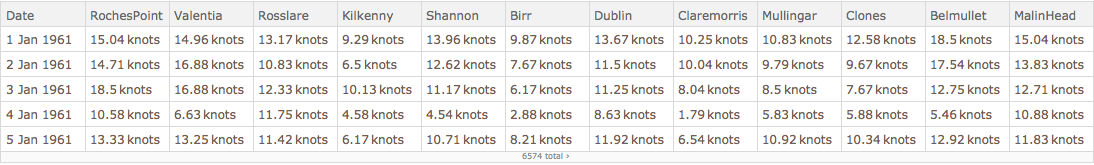### Analysis

Shuffle the dataset randomly and remove the unit labels:

 In:=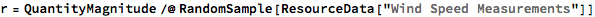Out=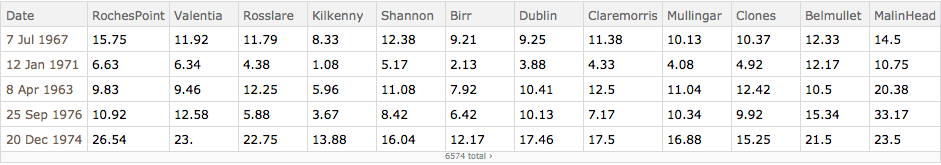Create a training dataset using 80% of the original dataset:

 In:=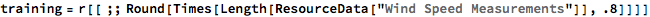Out=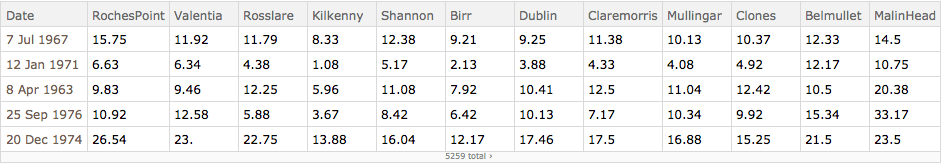Create a testing dataset using the remaining 20% of the original dataset:

 In:=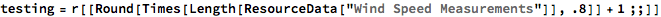Out=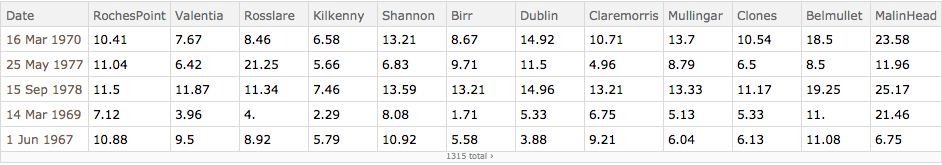Train a predictor function using "MalinHead" as the target variable:

 In:=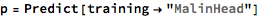Out=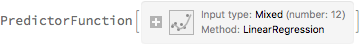Obtain general information about the predictor:

 In:=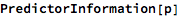Out=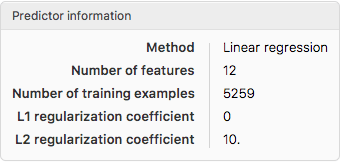Test the predictor:

 In:=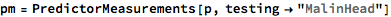Out=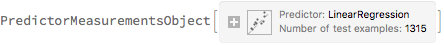Visualize the accuracy of the predictor:

 In:=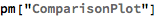Out=Wolfram Research, "Wind Speed Measurements" from the Wolfram Data Repository (2017) https://doi.org/10.24097/wolfram.63787.data